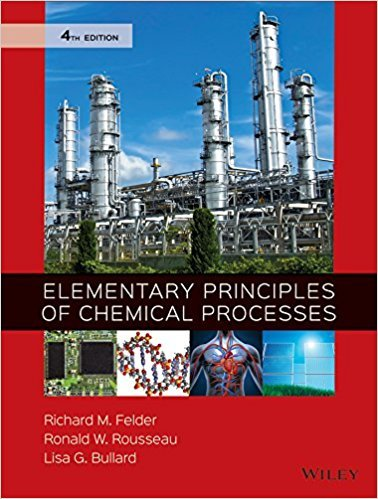×
×

# Solutions for Chapter 4.47: SUMMARY## Full solutions for Elementary Principles of Chemical Processes | 4th Edition

ISBN: 9780470616291Solutions for Chapter 4.47: SUMMARY

Solutions for Chapter 4.47
4 5 0 281 Reviews
16
3
##### ISBN: 9780470616291

Chapter 4.47: SUMMARY includes 1 full step-by-step solutions. This expansive textbook survival guide covers the following chapters and their solutions. Elementary Principles of Chemical Processes was written by and is associated to the ISBN: 9780470616291. This textbook survival guide was created for the textbook: Elementary Principles of Chemical Processes, edition: 4. Since 1 problems in chapter 4.47: SUMMARY have been answered, more than 45274 students have viewed full step-by-step solutions from this chapter.

Key Chemistry Terms and definitions covered in this textbook
• accuracy

A measure of how closely individual measurements agree with the correct value. (Section 1.5)

• alkaloids

Naturally occurring amines isolated from plants.

• allylic

The positions that are adjacent to the vinylic positions of a carboncarbon double bond.

• body-centered lattice

A crystal lattice in which the lattice points are located at the center and corners of each unit cell. (Section 12.2)

• chemical changes

Processes in which one or more substances are converted into other substances; also called chemical reactions. (Section 1.3)

• chemical reactions

Processes in which one or more substances are converted into other substances; also called chemical changes. (Section 1.3)

• conversion factor

A ratio relating the same quantity in two systems of units that is used to convert the units of measurement. (Section 1.6)

• critical pressure (Pc).

The minimum pressure necessary to bring about liquefaction at the critical temperature. (11.8)

• equilibrium-constant expression

The expression that describes the relationship among the concentrations (or partial pressures) of the substances present in a system at equilibrium. The numerator is obtained by multiplying the concentrations of the substances on the product side of the equation, each raised to a power equal to its coefficient in the chemical equation. The denominator similarly contains the concentrations of the substances on the reactant side of the equation. (Section 15.2)

• kinetic-molecular theory

A set of assumptions about the nature of gases. These assumptions, when translated into mathematical form, yield the ideal-gas equation. (Section 10.7)

• Mass spectrometry

An analytical technique for measuring the mass-to-charge ratio (m/z) of ions.

• mass-to-charge ratio(m/z)

Thedetermining factor by which ions are separatedfrom each other in mass spectrometry.

• miscible liquids

Liquids that mix in all proportions. (Section 13.3)

• Newman projection

A drawing style that is designed to show the conformation of a molecule.

• nuclear magnetic resonance (NMR)

A form of spectroscopy that involves the study of the interaction between electromagnetic radiation and the nuclei of atoms.

• oils

Triglycerides that are liquids at room temperature.

• optically active

Possessing the ability to rotate the plane of polarized light. (Section 23.4)

• polymer

A large molecule of high molecular mass, formed by the joining together, or polymerization, of a large number of molecules of low molecular mass. The individual molecules forming the polymer are called monomers. (Sections 12.1 and 12.8)

• random copolymer

A polymer, comprised of more than one kind of repeating unit, in which there is a random distribution of repeating units.

• solvolysis

A substitution reaction in which the solvent functions as the nucleophile.

×